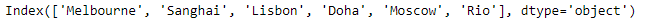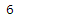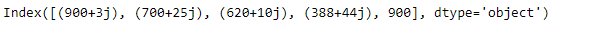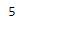Python | Pandas Index.size

• Last Updated : 20 Feb, 2019

Pandas Index is an immutable ndarray implementing an ordered, sliceable set. It is the basic object which stores the axis labels for all pandas objects.

Pandas Index.size attribute return the number of elements in the underlying data of the given Index object.

Syntax: Index.size

Parameter : None

Returns : number of elements in the Index

Example #1: Use Index.size attribute to find the number of elements in the underlying data of the given Index object.

 # importing pandas as pdimport pandas as pd  # Creating the indexidx = pd.Index(['Melbourne', 'Sanghai', 'Lisbon', 'Doha', 'Moscow', 'Rio'])  # Print the indexprint(idx)

Output :Now we will use Index.size attribute to find the number of elements in the given Index object.

 # return the number of elementsresult = idx.size  # Print the resultprint(result)

Output :As we can see in the output, the Index.size attribute has returned the number of elements in the given Index object.

Example #2 : Use Index.size attribute to find the number of elements in the underlying data of the given Index object.

 # importing pandas as pdimport pandas as pd  # Creating the indexidx = pd.Index([900 + 3j, 700 + 25j, 620 + 10j, 388 + 44j, 900])  # Print the indexprint(idx)

Output :Now we will use Index.size attribute to find the number of elements in the given Index object.

 # return the number of elementsresult = idx.size  # Print the resultprint(result)

Output :As we can see in the output, the Index.size attribute has returned the number of elements in the given Index object.

My Personal Notes arrow_drop_up